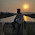Classification of Materials Based on Energy Band Structure

Based on the ability of various materials to conduct current, the materials are classified as conductors, insulators and the semiconductors.
A metal which is very good carrier of electricity is called conductor. A very poor conductor of electricity is termed as insulator. A metal having conductivity which is between conductor and an insulator is called semiconductor. Copper and aluminium are good examples of a conductor. Glass, wood, mica, diamond the examples of an insulator which do not conduct current. Silicon and germanium are the examples of a semiconductor which do not conduct current at low temperatures but as temperature increases these materials behave as good conductors. Let us see the energy band diagrams for these three types of metals.
1.1 Conductors
It has been mentioned earlier that a material having large number of free electrons can conduct very easily. For example, copper has 8.5 x 1028 free electrons per cubic metre which is a very large number. Hence copper is called good conductor. In fact, in metals like copper, aluminium there is no forbidden gap between valence band and conduction band. The two band overlap. Hence even at room temperature, a large number of electrons are available for conduction. So without any additional energy, such metals contain a large number of free electrons and hence called good conductors. An energy band diagram for a conductor is shown in the Fig. 1(a).
1.2 Insulators
An insulator has an energy band diagram as shown in the Fig. 1(b). In case of such insulating material, there exists a large forbidden gap in between the conduction band and the valence band. Practically it is impossible for an electron to jump from the valence band to the conduction band. Hence such materials can not conduct and called insulators. The forbidden gap is very wide, approximately of about 7 eV is present in insulators. For a diamond, which is an insulator, the forbidden gap is about 6 eV. Such materials may conduct only at very high temperatures or if they are subjected to high voltage. Such a conduction is rare and is called breakdown of an insulator. The other insulating materials are glass, wood, mica, paper etc.Fig. 1 Energy band diagrams

1.3 Semiconductors
Now let us come to an important category of materials, which are neither insulators nor conductors. The forbidden gap in such materials is very narrow as shown in Fig. 1(c). Such materials are called semiconductors. The forbidden gap is about 1 eV. In such materials, the energy provided by the heat at room temperature is sufficient to lift the electrons from the valence band to the conduction band. Therefore at room temperature, semiconductors are capable of conduction. But a 0o K or absolute zero (-273o C), all the electrons of semiconductor materials find themselves locked in the valence band. Hence at 0o K, the semiconductor materials bahave as perfect insulators. In case of semiconductors, forbidden gap energy depends on the temperature. For silicon and germanium, this energy is given by,

where T = Absolute temperature in K
Assuming room temperature ti be 27o C i.e. 300o K, the forbidden gap energy for Si and Ge can be calculated from the above equations. The forbidden gap for the germanium is 0.72 eV while for the silicon it is 1.12 eV at room temperature. The silicon and germanium are the two widely used semiconductor materials in electrons devices, as mentioned earlier.
key Point : While calculating , substitute T in ok.
Why silicon is most widely used ?
Looking at the structure of silicon and germanium atom, it can be seen that valence shell of silicon is 3rd shell while valence shell of germanium is 4rd shell. Hence valence electrons of germanium are at larger distance from nucleus than valence electrons of silicon. Hence valence electrons of germanium are more loosely bound to the nucleus than those of silicon. Thus valence electrons of germanium can easily escape from the atom, due to very small additional energy imparted to them. So at high temperature, germanium becomes unstable than silicon and hence silicon is widely used semiconductor material.
Example : Calculate the value of forbidden gap for silicon and germanium at the temperature of 35o C.
Solution : Forbidden gap for silicon is given by,
EG   = 1.12 - 3.6 x 10 x T
Now    T = 35 + 273 = 308 oK
...                EG   = 1.21 - 3.6 x 10-4 x 308 = 1.099 eV
While forbidden gap for germanium is given by,
EG   = 0.785 - 2.23 x 10-4 x T = 0.785 - 2.23 x 10-4 x 308 = 0.7163 eV

1.2.3.